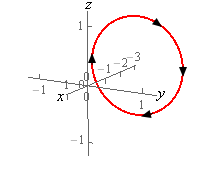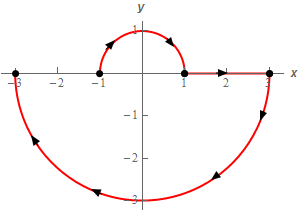Paul's Online Notes
Home / Calculus III / Line Integrals / Line Integrals - Part I
Show Mobile Notice Show All Notes Hide All Notes
Mobile Notice
You appear to be on a device with a "narrow" screen width (i.e. you are probably on a mobile phone). Due to the nature of the mathematics on this site it is best views in landscape mode. If your device is not in landscape mode many of the equations will run off the side of your device (should be able to scroll to see them) and some of the menu items will be cut off due to the narrow screen width.
Assignment Problems Notice
Please do not email me to get solutions and/or answers to these problems. I will not give them out under any circumstances nor will I respond to any requests to do so. The intent of these problems is for instructors to use them for assignments and having solutions/answers easily available defeats that purpose.

If you are looking for some problems with solutions you can find some by clicking on the "Practice Problems" link above.

### Section 16.2 : Line Integrals - Part I

For problems 1 – 10 evaluate the given line integral. Follow the direction of $$C$$ as given in the problem statement.

1. Evaluate $$\displaystyle \int\limits_{C}{{3y\,ds}}$$ where $$C$$ is the portion of $$x = 9 - {y^2}$$ from $$y = - 1$$ and $$y = 2$$.
2. Evaluate $$\displaystyle \int\limits_{C}{{\sqrt x + 2xy\,ds}}$$ where $$C$$ is the line segment from $$\left( {7,3} \right)$$ to $$\left( {0,6} \right)$$.
3. Evaluate $$\displaystyle \int\limits_{C}{{{y^2} - 10xy\,ds}}$$ where $$C$$ is the left half of the circle centered at the origin of radius 6 with counter clockwise rotation.
4. Evaluate $$\displaystyle \int\limits_{C}{{{x^2} - 2y\,ds}}$$ where $$C$$ is given by $$\vec r\left( t \right) = \left\langle {4{t^4},{t^4}} \right\rangle$$ for $$- 1 \le t \le 0$$.
5. Evaluate $$\displaystyle \int\limits_{C}{{{z^3} - 4x + 2y\,ds}}$$ where $$C$$ is the line segment from $$\left( {2,4, - 1} \right)$$ to $$\left( {1, - 1,0} \right)$$.
6. 6. Evaluate $$\displaystyle \int\limits_{C}{{x + 12xz\,ds}}$$ where $$C$$ is given by $$\displaystyle \vec r\left( t \right) = \left\langle {t,\frac{1}{2}{t^2},\frac{1}{4}{t^4}} \right\rangle$$ for $$- 2 \le t \le 1$$.
7. Evaluate $$\displaystyle \int\limits_{C}{{{z^3}\left( {x + 7} \right) - 2y\,ds}}$$ where $$C$$ is the circle centered at the origin of radius 1 centered on the $$x$$-axis at $$x = - 3$$ . See the sketches below for the direction.8. Evaluate $$\displaystyle \int\limits_{C}{{6x\,ds}}$$ where $$C$$ is the portion of $$y = 3 + {x^2}$$ from $$x = - 2$$ to $$x = 0$$ followed by the portion of $$y = 3 - {x^2}$$ form $$x = 0$$ to $$x = 2$$ which in turn is followed by the line segment from $$\left( {2, - 1} \right)$$ to $$\left( { - 1, - 2} \right)$$. See the sketch below for the direction.9. Evaluate $$\displaystyle \int\limits_{C}{{2 - xy\,ds}}$$ where $$C$$ is the upper half of the circle centered at the origin of radius 1 with the clockwise rotation followed by the line segment form $$\left( {1,0} \right)$$ to $$\left( {3,0} \right)$$ which in turn is followed by the lower half of the circle centered at the origin of radius 3 with the clockwise rotation. See the sketch below for the direction.10. Evaluate $$\displaystyle \int\limits_{C}{{3xy + {{\left( {x - 1} \right)}^2}\,ds}}$$ where $$C$$ is the triangle with vertices $$\left( {0,3} \right)$$, $$\left( {6,0} \right)$$ and $$\left( {0,0} \right)$$ with the clockwise rotation.
11. Evaluate $$\displaystyle \int\limits_{C}{{{x^5}\,ds}}$$ for each of the following curves.
1. $$C$$ is the line segment from $$\left( { - 1,3} \right)$$ to $$\left( {0,0} \right)$$ followed by the line segment from $$\left( {0,0} \right)$$ to $$\left( {0,4} \right)$$.
2. $$C$$ is the portion of $$y = 4 - {x^4}$$ from $$x = - 1$$ to $$x = 0$$.
12. Evaluate $$\displaystyle \int\limits_{C}{{3x - 6y\,ds}}$$ for each of the following curves.
1. $$C$$ is the line segment from $$\left( {6,0} \right)$$ to $$\left( {0,3} \right)$$ followed by the line segment from $$\left( {0,3} \right)$$ to $$\left( {6,6} \right)$$.
2. $$C$$ is the line segment from $$\left( {6,0} \right)$$ to $$\left( {6,6} \right)$$.
13. Evaluate $$\displaystyle \int\limits_{C}{{{y^2} - 3z + 2\,ds}}$$ for each of the following curves.
1. $$C$$ is the line segment from $$\left( {1,0,4} \right)$$ to $$\left( {2, - 1,1} \right)$$.
2. $$C$$ is the line segment from $$\left( {2, - 1,1} \right)$$ to $$\left( {1,0,4} \right)$$.